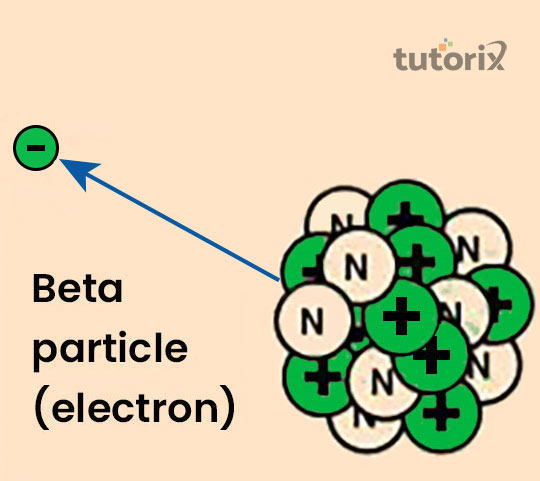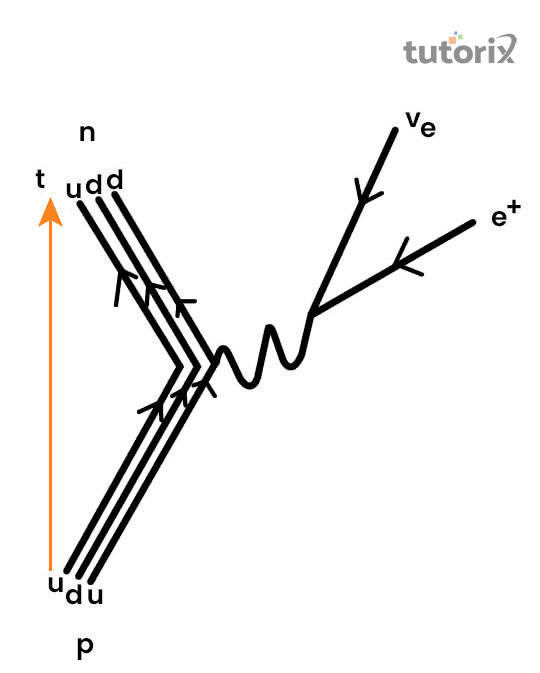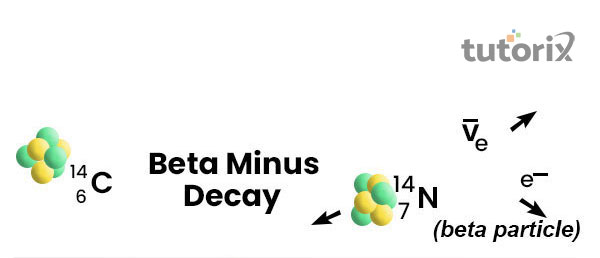## Introduction

The “radioactive beta decay” process exists in the field of nuclear physics. The radioactive process of decay is associated with the process of reaction in which beta ray is released from the “nucleus of an atom”.

It helps in the transformation of the “original nuclides” to isobars” of the nuclide. In simple terms, in the process of “beta decay”, the “neutron” transforms into a “proton” through the “emission of an electron” which is further accompanied by “antineutrino”.

The process of “beta decay” is resultant of a weak force which makes the “decay process” a relatively lengthy process.

## What is “radioactivity beta decay”?

The “radioactivity beta decay” process is associated with the process of emission of a beta ray in a “nuclear reaction”. According to Pommé et al. (2018), in this process, a “proton” is transformed into a “neutron” and this process occurs inside the “nucleus” of a “particular radioactive sample”. The “beta decay” process helps the nucleus of a particular “radioactive sample” to attain a similar state of an “optimum neutron/proton ratio” and in this process; a beta particle is released (phy-astr, 2022). The “beta particles” can either be “electrons” or “positrons”. The “process of beta decay” occurs due to “weak interaction”.Figure 1: Beta decay

## What happens in the process of “radioactivity Beta Decay”?

• The process of “beta decay” is a “radioactive process”, in this process; a “beta ray” is generated from the “nucleus of an atom”. In the process of “beta decay”, a “proton” in the “nucleus” transforms into a “neutron” or the reverse process might occur as well.
• According to Parkhomov (2018), this leads to the two separate processes, in case the “proton” is “turned into neutron”, the process is termed “β+ decay” and similarly in case, a “neutron” is “converted to proton” the process is called “β- decay”.
• According to Algora et al. (2021), this change in the condition of the “nucleus” causes an “emission” of a “beta particle”. In the case of “β+ decay”, the “beta particle” is a “positron” and in the case of “β- decay” the particle is a “high-speed electron.## Beta-decay: example

“Beta decay” of “carbon atoms” is one of the primary examples of this process. In this case, a “neutron” of carbon is “turned into a proton” and thereby the emitted “beta particle” is an “electron”. Similarly, in the case of “β+ decay of carbon-10”, the “proton of the carbon atom” is “turned into a neutron” and the “beta particle” that is released is a “positron”.

## Different types of “Beta decay”

The “radioactive beta decay” process is classified into two categories,

• “beta-minus decay” and
• “beta-plus decay”.

In the case of “beta-minus decay”, a particular “neutron” is transformed to generate “a proton” and it leads to a rise in the “atomic number”. The “neutron is neutral” and the “proton is positive”. In order “to maintain the conservation of charge”, the “nucleus” involved in the process also helps in the production of an “electron” and an “anti-neutrino” (lbl, 2022). “Anti-neutrino” is the “antimatter” counterpart of “neutrino”. These two are neutral and these two particles also possess a negligible amount of mass. These particles have a very “weak interaction” with the matter and can even pass through the earth without being bothered. Examples of “beta-minus decay" includes the conversion of 14C to 14N.Figure 3: Beta-minus decay

In the case of “beta-plus decay” the “proton” breaks down to generate a “neutron” and it causes a significant reduction in the “atomic number” of the given “radioactive sample”. The “nucleus” loses “protons” but it gains “electrons” in the process. The “conservation of charge” is an important factor in this process of “radioactive decay”. The “beta-plus decay” generates a “positron” and a “neutrino” to obey the ‘conservation of charge” (atomicarchive, 2022). A “positron” is the anti-matter of the “electro” and it has similar properties as well except “positron” is positively charged. In the process of “beta-decay”, the behaviour of a “neutrino” is similar to the “antineutrinos”.

## What is “Fermi’s theory” for “radioactivity beta decay”?

“Fermi’s theory” of “radioactivity beta decay” is also called “Fermi’s interaction”. He was the first person to invent the “world’s first nuclear reactor”. He stated that “four fermions” interact directly with one another at a “single vortex” and this interaction explains the process of “beta decay” by direct coupling of “neutron” with an “electron” and coupling occurs between “neutrino” and “proton”.

## Conclusion

The “radioactive beta decay” process deals with a set of “nuclear reactions” in which a “neutron” decomposes into a “proton” and an “electron”. This process helps in the attainment of a stable “ratio of proton and neutron” for the unstable atoms. This process is a direct consequence of a relatively “weak force of interaction”.

## FAQs

Q1. What is the condition for “beta plus decay”?

Ans. “Beta plus decay” happens only in case the “daughter nucleus” is more stable compared to the “mother nucleus”. This difference refers to the “conversion of proton” into a “neutron” or a “positron” into a neutrino”.

Q2. Which particles are called “beta particles”?

Ans. In “beta decay”, the “beta particle” is referred to as the “high-speed electron” when it undergoes “beta-minus decay” or a “positron” when it undergoes a “beta-plus decay”. In this process, a “neutron” of a “carbon atom” is turned into a “proton” and the resultant emitted “beta particle” is an “electron”.

Q3. Does the “radioactive beta decay” process release energy?

Ans. Yes, in the course of “radioactive beta decay” a significant amount of “kinetic energy” is released. The value typically ranges from “0 to 1MeV”, the value, however, may range from a few “KeV” to “tens of Mev”

Q4. Who discovered the process of beta decay?

Ans. Enrico Fermi illustrated the process of “beta decay” in 1933. This process is called “Fermi's theory of beta decay” or “Fermi's interaction”.

Q5. What is the process of “electron capture”?

Ans. In this process, an "electron" is captured by a "proton" in the nucleus and an "electron neutrino" is released. This process is associated with “beta decay” for its “nuclear process” is governed by a weak force.

## References

### Journals

Algora, A., Tain, J. L., Rubio, B., Fallot, M., & Gelletly, W. (2021). Beta-decay studies for applied and basic nuclear physics. The European Physical Journal A, 57(3), 1-28.Retreievd from: https://link.springer.com/article/10.1140/epja/s10050-020-00316-4

Parkhomov, A. G. (2018). Rhythmic and Spоradic Changes in the Rate of Beta Decays: Possible Reasons. Journal of Modern Physics, 9(8), 1617-1632.Retrieved from: https://www.scirp.org/journal/paperinformation.aspx?paperid=86108

Pommé, S., Stroh, H., Altzitzoglou, T., Paepen, J., Van Ammel, R., Kossert, K., ... & Bruggeman, M. (2018). Is decay constant?. Applied Radiation and Isotopes, 134, 6-12. Retrieved from: https://www.sciencedirect.com/science/article/pii/S0969804317303822